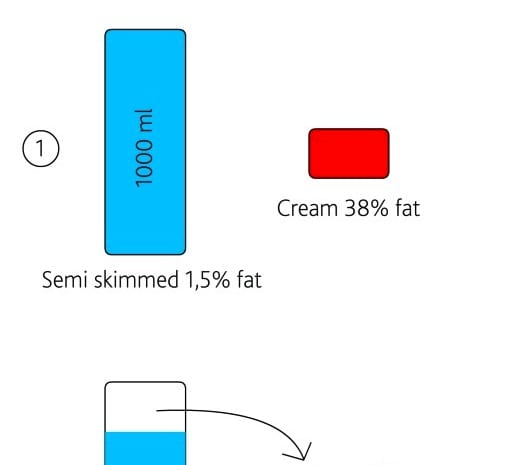# Customize the fat percentage in your milk

First a warning:

This is geeky, slightly – if at all – useful. It might be just me, but I think its fun..

Baristas play with all sorts of parameters. Why not play with the fat percentage of the milk? I have developed a method and a accompanying formula you can use.

In the example below I have used a Danish semi skimmed milk that contain 1,5% fat and a Danish cream with 38% fat (1 in the figure).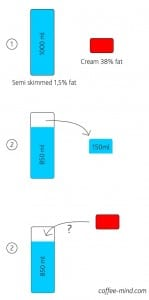The procedure is as follows: Take a liter of the semi skimmed milk and pour out exactly 150 mL (figure 2) to make room for addition of cream. Decide the fat percentage you would like in your milk. In the formula below you can find the amount of cream that you need to add to the 850 mL milk left in the milk carton in order to reach the desired fat percentage (figure 3). The formula that will give you the right answer: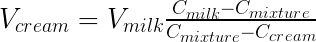which with the above products (skimmed milk with 1,5% fat and cream with 38% fat) gives: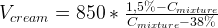So now you can just insert the fat percentage you would like in formula above where it says Cmixture

Say you happen to live in a country where a semi skimmed milk has 1,2 % fat and a cream has 42% fat, and for some reason you would like to adjust the milk to 3,2 % fat. In that case you  can insert the numbers in the formula as follows: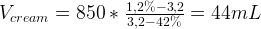So using the formula we have found out that we need to ad 44 mL of cream to the semi skimmed milk in the container in order to end up with a milk with 3,2 % fat.

UPDATE: Jason Stewart suggested this service to do the calculations: www.symbolab.com

Geeky and slightly useful. I know.

If you would like to go a step deeper, here is how the formula is developed:

Obvious parameters in the problem:

Cmixture: concentration of fat in the mixture of milk and cream
Cmilk: concentration of fat in the milk
Ccream: concentration of fat in the cream
Vmilk: volume of milk
Vcream: volume of cream

So how do we put them into relation, so that we can get Vcream isolated in the formula so that it can be calculated as a function of the other parameters?

A concentration is the amount of something per volume and in the case of milk and cream it is the amount of fat i grams per liter. Expressing ‘F’ as the amount of fat we could put up these that will come in handy in a while: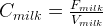and this could be rewritten into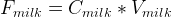and the same relation goes for cream: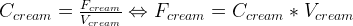So what do we know? We know, that the total amount of fat in the mixture is the sum of the fat in milk and cream: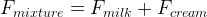And it should be obvious that the total volume of the mixture is the volume of milk and cream put together: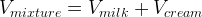And the concentration of the mixture is the total amount of fat (as indicated above) divided by the total volume of the mix (also indicated above) and mathematically this claim looks like this: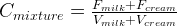We don’t know Fmilk and Fcream directly but notice how I above calculated F for both milk and cream by multiplying concentration and volume so if we put that into the equation we get this: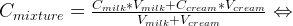So now we have all the important parameters put into a mathematical relationship and therefore solved the problem conceptually and the solution is now implicit. We can make it explicit by isolating Vcream on the left side by doing some classical school math: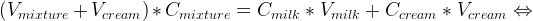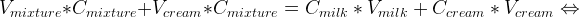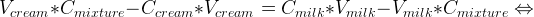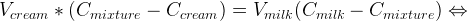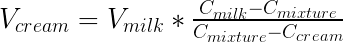Which solves the problem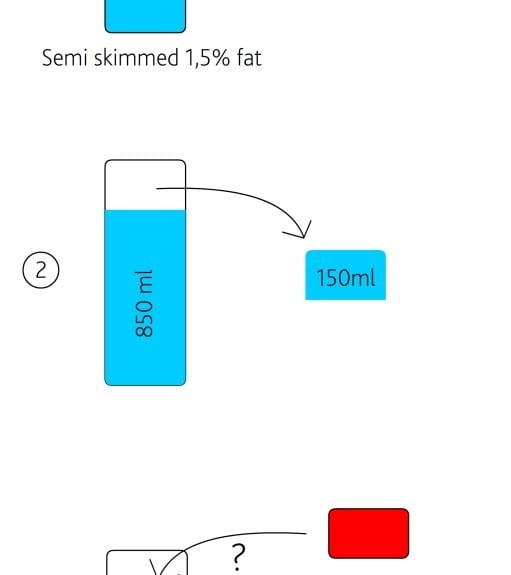#### Morten Münchow

Founder of CoffeeMind. Coffee roaster, educator/trainer and researcher. Read more here

1.Melissa says:

I love this; it’s exactly what I was looking for.
I plan to use the formula to make yogurt of different fat percentages.

2.Julia says:

What amounts of sugar, protein, carbohydrates and fibre are in the Danish cream you used?

1.Morten Münchow says:

Dear Julia

I don’t think there is any fiber in Danish cream 🙂

These are the ingredients in the particular cream used:
Fat 38%
Carbs 3,2%
Protein 2,1%
Salt 0,07%
Energy 1496 kJ / 363 kcal

3.Dave says:

To me, it seems like the leftmost “Vmixtures” in the first two lines of the final derivation of the result equation should be “Vmilk”